﻿ Ward’s Generalized Special FunctionsPublications are Open
Access in this journal
Article Versions
Export Article
• Normal Style
• MLA Style
• APA Style
• Chicago Style
Research Article
Open Access Peer-reviewed

Ward’s Generalized Special Functions

Anthony G. Shannon , Ömür Deveci
Turkish Journal of Analysis and Number Theory. 2018, 6(3), 90-92. DOI: 10.12691/tjant-6-3-4
Received January 03, 2018; Revised February 26, 2018; Accepted June 16, 2018

Abstract

This paper considers generalizations of Bernoulli and Euler numbers to clarify and extend some known relations studied by Morgan Ward. It does this with the Euler-Maclaurin sum formula. It relates the mappings to category theory as a means of applying the ideas further.

1. Introduction

We explore here some formal aspects of generalized Bernoulli numbers in terms of normal divisibility sequences as defined by Morgan Ward 1, 2. The generalized Bernoulli polynomials in question have the form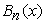such that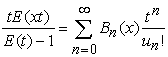(1)

in which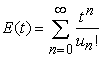(2)

is an analogous Euler function and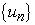is a sequence which is normal in that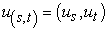and divisible in that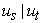whenever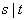. We shall also require that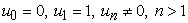and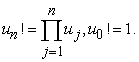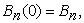a generalized Bernoulli number. Ward’s generalized coefficients were actually rediscoveries of work by Fontené 3.

2. Associated Operators

We use the operator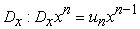, and forward difference operator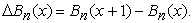(3)

Formally,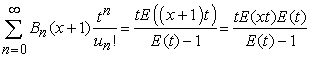so that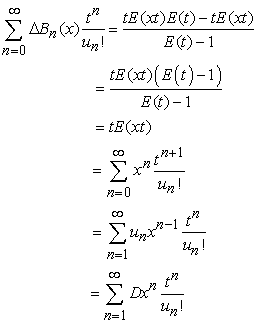and so that on equating coefficients of t, we get the rather neat result that extends Ward 4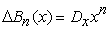(4)

3. Generalized Bernoulli Numbers

As with ordinary Bernoulli numbers we set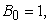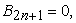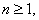and define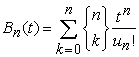(5)

where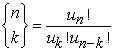is a Fontené-Ward binomial coefficient for which Henry Gould 3 obtained some elegant results. (For other approaches to generalized integers see Graeme Cohen 5.) The generalization used here consists essentially in systematically replacing the ordinary binomial coefficient with a binomial coefficient to the base u. Thus, when {un} = Z, the set of integers, the two binomial coefficients are formally identical. We shall refer briefly to the mappings in Section 5. When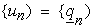, the set of Fermatian numbers 6 defined by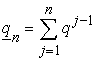where q may be indeterminate with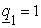, we get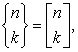the well-known q-binomial coefficient 7. Note that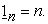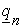is described as the nth Fermatian of index q 8. More particularly, when {un} is the sequence of Fibonacci numbers we have the Fibonacci binomial coefficients 9. Ward has defined a sequence to be normal when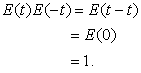So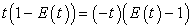and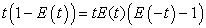so that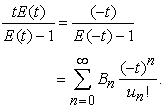But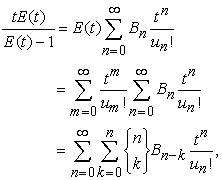and so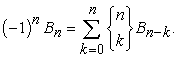(6)

Sincewhen {un} = Z, these reduce to the ordinary Bernoulli numbers 10.

4. Euler-Maclaurin Formula

The importance of the ordinary Bernoulli numbers comes primarily from the Euler-Maclaurin sum-formula for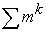. A generalization of this is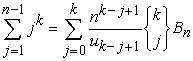(7)

the proof of which follows: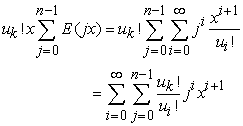and the coefficients of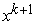are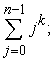since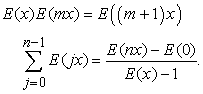Thus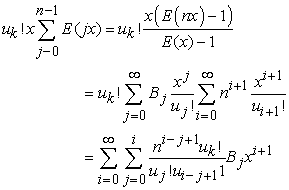and the coefficients of xk+1 are as required in equation (7).

Hence, these generalized Bernoulli numbers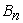and the ordinary Bernoulli numbers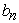are actually related by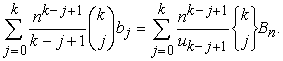(8)

The Euler-Maclaurin formula is also of interest within the context of this paper because it is used in the classic proof of the Staudt-Clausen Theorem by Richard Rado 10, 11 and its extensions 12, 13.

Further related research can be carried out with applications of the q-umbral calculus 14 or with category theory. For an example of the latter, let u be the sequence function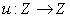so that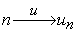; let h represent the highest common factor function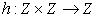and then we have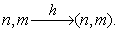The divisibility sequences mentioned in this paper can then be represented in terms of a commutative diagram: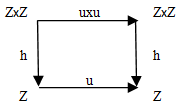For example,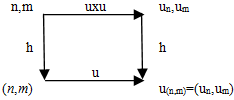Now a commutative diagram of four sets and functions with such a composition of functions determines a concrete category. It would be of interest to seek a natural transformation of functions between this and other categories either to generalize or to clarify the structure of the number theoretic results. The theory of categories has been applied to the problem in psychology of abstraction from the senses to the intellect through perception 15 by one of the present writers with Anthony Allen.

Gratitude is expressed to an anonymous referee for several corrections to the original manuscript.

References

  Ward, Morgan, “Divisibility sequences,” Bulletin of the American Mathematical Society, 42, 843-8455, 1936. In article View Article  Ward, Morgan, “Memoir on elliptic divisibility sequences,” American Journal of Mathematics, 70 (1), 31-74, 1948. In article View Article  Gould, H.W., “The bracket-function and Fontené-Ward generalized binomial coefficients with applications to fibonomial coefficients,” The Fibonacci Quarterly, 7 (1), 23-40, 55, 1969. In article View Article  Ward, Morgan. “A calculus of sequences,” American Journal of Mathematics, 58, 255-266, 1936. In article View Article  Cohen, G.L., “Selberg formulae for Gaussian integers,” Acta Arithmetica, 16, 385-400, 1975. In article View Article  Dickson, L.E. History of the Theory of Numbers, Volume 1. New York, Chelsea, Ch.XVI, 1952. In article  Carlitz, L. and Riordan, J., “Two element lattice permutation numbers and their q-generalization,” Duke Mathematical Journal, 31, 371-388, 1964. In article View Article  Shannon, A.G., “Some Fermatian Special Functions,” Notes on Number Theory and Discrete Mathematics, 9 (4), 73-82, 2003. In article View Article  Hoggatt, Verner E. Jr., “Fibonacci numbers and generalized binomial coefficients,” The Fibonacci Quarterly, 5, 383-400, 1967. In article View Article  Rado, R., “A new proof of a theorem of v. Staudt,” Journal of the London Mathematical Society, 9 (1), 85-88, 1934. In article View Article  Rado, R., “A note on the Bernoullian numbers,” Journal of the London Mathematical Society, 9 (1), 88-90, 1934. In article View Article  Carlitz, L., “The Staudt-Clausen theorem,” Mathematics Magazine, 35, 131-146, 1961. In article View Article  Horadam, A.F. and Shannon, A.G.,“Ward’s Staudt-Clausen problem,” Mathematica Scandinavica, 29 (4), 239-250, 1976. In article View Article  Roman, Steven, The Umbral Calculus, Orlando, FL: Academic Press, Ch.6, 1984. In article  Allen, A.L. and Shannon, A.G., “Note on a category model for an abstraction mechanism.” Psychological Reports. 27: 591-594, 1970. In article View Article

Published with license by Science and Education Publishing, Copyright © 2018 Anthony G. Shannon and Ömür DeveciThis work is licensed under a Creative Commons Attribution 4.0 International License. To view a copy of this license, visit http://creativecommons.org/licenses/by/4.0/